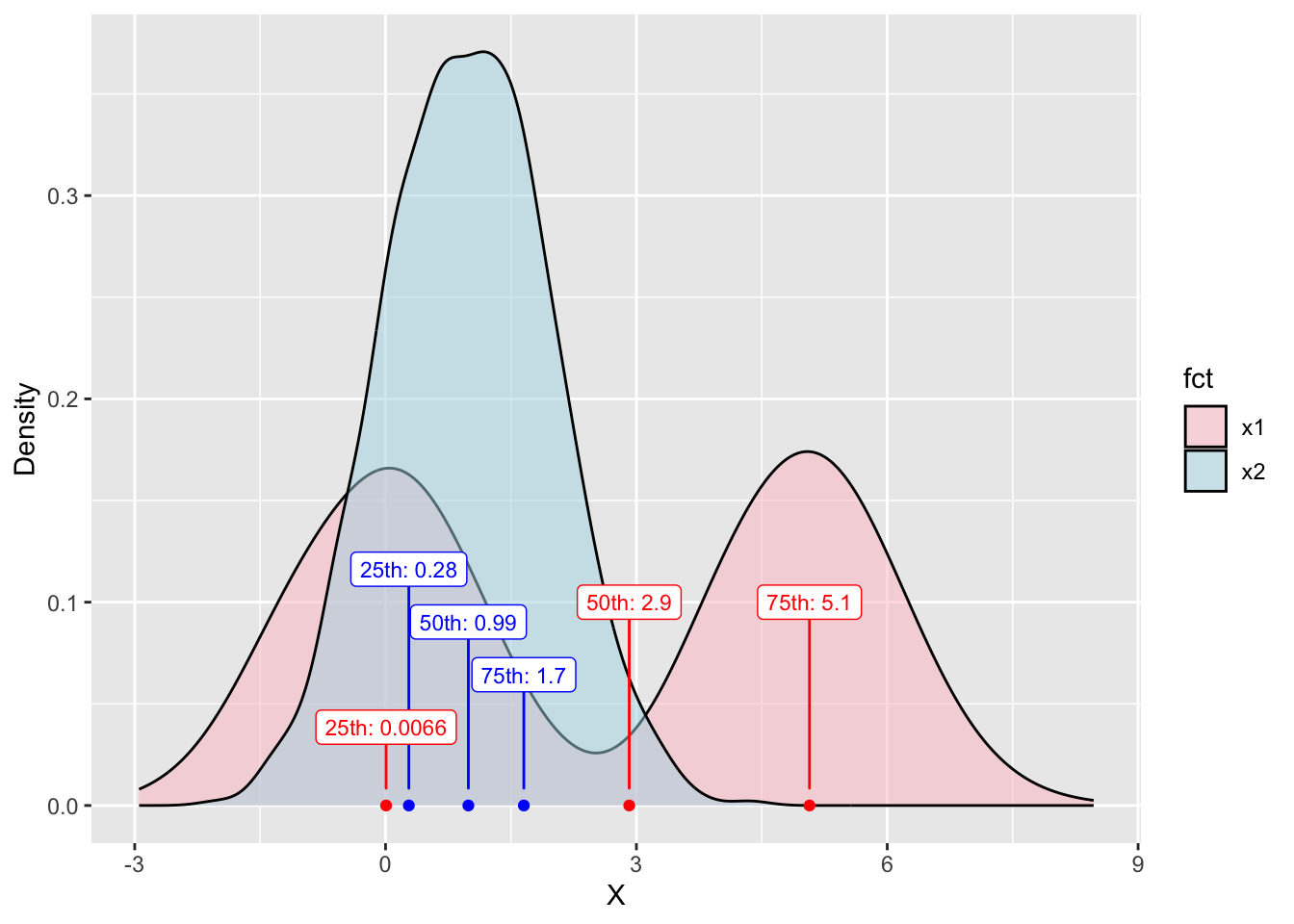An R implementation of https://www.ncbi.nlm.nih.gov/pmc/articles/PMC4811631/. This answers the question: the Wald test compares population medians, but what about other parts of the distribution. Johnson and Romer provided and algorithm to compare a limited set of percentiles between two distributions. I created a function Wald_percentiles_test() to implement it, code is on Github.

source('wald_percentiles_test.R')

# population 1: bimodal
x1 <- c(rnorm(500, mean = 0, sd = 1), rnorm(500, 5, 1))

# pooulation 2: normal
# note: can be a different length!
x2 <- c(rnorm(800, mean = 1, sd = 1))

# percentiles to test
p <- c(0.25, 0.50, 0.75)

# number of bootstrap runs
n.B <- 500

Wald_percentiles_test(x1, x2, p, n.B)## W > qchisq?  TRUE * Differences btwn percentile profiles are statistically significant
## CI95 of diff at p= 0.25 : [ -0.67 ,  0.12 ]
## CI95 of diff at p= 0.5 : [ -0.54 ,  4.4 ]
## CI95 of diff at p= 0.75 : [ 3 ,  3.8 ]  * Significant difference